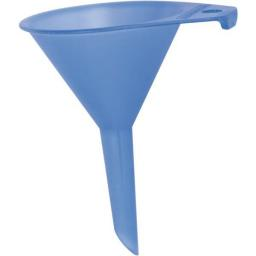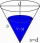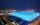# Funnel

The funnel has the shape of an equilateral cone. Calculate the surface wetted with water if we poured into the funnel 7.1 liters of water.

S =  15.6 dm2

### Step-by-step explanation:Did you find an error or inaccuracy? Feel free to write us. Thank you!Tips to related online calculators

#### You need to know the following knowledge to solve this word math problem:

We encourage you to watch this tutorial video on this math problem:

## Related math problems and questions:

• The funnelThe funnel has the shape of an equilateral cone. Calculate the content of the area wetted with water if you pour 3 liters of water into the funnel.
• Cube 6The surface area of one wall cube is 1600 cm square. How many liters of water can fit into the cube?
• Right circular coneThe volume of a right circular cone is 5 liters. Calculate the volume of the two parts into which the cone is divided by a plane parallel to the base, one-third of the way down from the vertex to the base.
• Equilateral cylinderEquilateral cylinder (height = base diameter; h = 2r) has a volume of V = 199 cm3 . Calculate the surface area of the cylinder.
• Axial sectionThe axial section of the cone is an equilateral triangle with area 168 cm2. Calculate the volume of the cone.
• Big cubeCalculate the surface of the cube, which is composed of 64 small cubes with an edge 1 cm long.
• Cube-shaped boxThe cube-shaped box is filled to the brim with 2 liters of milk. Calculate the edge and surface of the box.
• Cube and waterHow many liters of water can fit into a cube with an edge length of 0.11 m?
• Calculate 3Calculate the cube volume whose edge is 3x-1,3x-1,3x-1
• Equilateral coneWe pour so much water into a container that has the shape of an equilateral cone, the base of which has a radius r = 6 cm, that one-third of the volume of the cone is filled. How high will the water reach if we turn the cone upside down?
• Cube into cylinderIf we dip a wooden cube into a barrel with a 40cm radius, the water will rise 10 cm. What is the size of the cube edge?
• Chemical parisonThe blown parison (with shape of a sphere) have a volume 1.5 liters. What is its surface?
• CubeOne cube has an edge increased five times. How many times will larger it's surface area and volume?
• Cube surfce2volumeCalculate the volume of the cube if its surface is 150 cm2.
• Cylinder - areaThe diameter of the cylinder is one-third the length of the height of the cylinder. Calculate the surface of cylinder if its volume is 2 m3.
• TerezaThe cube has an area of base 256 mm2. Calculate the edge length, volume, and area of its surface.
• The cubeThe cube has a surface area of 486 m ^ 2. Calculate its volume.